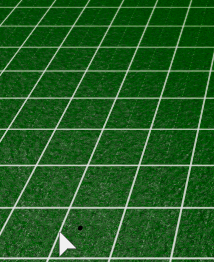# Snapping to Grid

If anyone can explain the concept, thatd be great

Well i am attempting to snap to Grid but there is an odd offset depending on the position of the Grid itself

I cant think of any solutions to attempthere is how it snaps as of now

``````local GridObject = player.Name.."'s Grid"
local Structure = GridModel.Structure
local Grid = GridModel[GridObject]
local GridSize = 3
--local Offset = .2
local Marker = game.Workspace.Marker

Marker.CFrame = CFrame.new((math.floor(mouse.Hit.X / GridSize +.5 ) * GridSize), Grid.Position.Y + .1, (math.floor(mouse.Hit.Z / GridSize ) * GridSize))`````````
1 Like

I am going to assume that this grid is a texture on a block.
Have you tried changing the `StudsPerTileU` and `StudsPerTileV` properties of the Texture so that it fits? The formula for `Marker.CFrame` looks perfect except for adding 0.5 to the formula of the Z component of the CFrame:

``````Marker.CFrame = CFrame.new(
(math.floor(mouse.Hit.X / GridSize +.5 ) * GridSize),
Grid.Position.Y + .1,
(math.floor(mouse.Hit.Z / GridSize +.5 ) * GridSize)
-- The .5 in math.floor() was missing
)
``````

How did you do that codeblock?

Try formatting your code using three tick marks or three squiglies:

Input:
```
--code here
```

Output:

``````--code here
``````

This will also work:
~~~
--code here
~~~

Now it does snap but it doesnt snap according to the Grid
like if the Grid was snapping properly then I were to move the grid slightly up there would be an offset

``````function Round(x, mult)
return math.floor((x / mult) + 0.5) * mult
end
``````

Where `x` is your input and `mult` is your grid size. I use this very code for games to lock to a grid.

6 Likes

still didnt work i used the function

``````function Round(x, mult)

return math.floor((x / mult) + 0.5) * mult

end
``````
``````Marker.CFrame = CFrame.new(Round(mouse.Hit.X, GridSize) , Grid.Position.Y + .1, Round(mouse.Hit.Z, GridSize))
``````

Could I get an image of what your marker is currently doing, similar to what you have in the original post?

If you want your grid to line up properly:

• `GridSize` has to be the same as `StudsPerTile` on the texture (obviously)
• Position and Size of the parent part both have to be a multiple of 6 on the X and Z axes, I believe? It might just be a multiple of 3.
• I think Position has to be a multiple of the `GridSize` on X and Z, and Size has to be a multiple of `2 * GridSize` on X and Z as well.

And that should be it.

You can just round the grid part off using @Crazyman32’s function:

``````Grid.Position = Vector3.new(
Round(Grid.Position.X, GridSize),
Grid.Position.Y,
Round(Grid.Position.Z, GridSize)
)

Grid.Size = Vector3.new(
Round(Grid.Size.X, 2 * GridSize),
Grid.Size.Y,
Round(Grid.Size.Z, 2 * GridSize)
)
``````
2 Likes